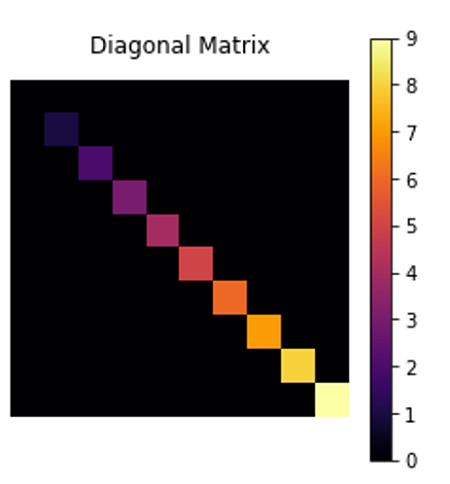# Python | Drawing Diagonal Matrix using Matplotlib

In this tutorial, we are going to learn how to draw a diagonal matrix in python using matplotlib?
Submitted by Anuj Singh, on August 05, 2020

Matplotlib provides an inbuilt function for plotting matrices i.e. matplotlib.pyplot.matshow(). We first define a diagonal matrix using numpy library function numpy.diag() and then plot it using matplotlib. There are a number of color maps available and for illustration, we are using inferno and cool color maps in this article.

Illustration:## Python code for drawing diagonal matrix using matplotlib

```import matplotlib.pyplot as plt
import numpy as np

a = np.diag(range(10))
plt.matshow(a)
plt.axis(False)
plt.cool()
plt.title('Diagonal Matrix')
plt.colorbar()
plt.show()

plt.matshow(a)
plt.inferno()
plt.axis(False)
plt.title('Diagonal Matrix')
plt.colorbar(label='mat')
plt.show()
```

Output:

```Output is as figure
```

Languages: » C » C++ » C++ STL » Java » Data Structure » C#.Net » Android » Kotlin » SQL
Web Technologies: » PHP » Python » JavaScript » CSS » Ajax » Node.js » Web programming/HTML
Solved programs: » C » C++ » DS » Java » C#
Aptitude que. & ans.: » C » C++ » Java » DBMS
Interview que. & ans.: » C » Embedded C » Java » SEO » HR
CS Subjects: » CS Basics » O.S. » Networks » DBMS » Embedded Systems » Cloud Computing
» Machine learning » CS Organizations » Linux » DOS
More: » Articles » Puzzles » News/Updates